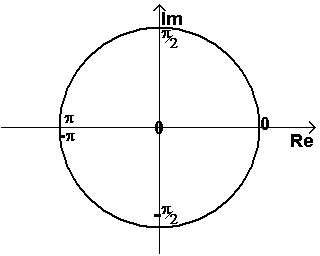### Predefined Functions – atan2

 Function name: atan2 – Arcus Tangent (angle) Synopsis: `atan2(x)`

Input data type Output data type
real real
complex real
quaternion unsupported

Description:

atan2 and arg are the same functions. If the input value is real, then it first gets converted to a complex number by setting the imaginary value to 0. Positive real values lead to 0, negative real values lead to p.

This function calculates the angle of the complex number. The result is between -p (exclusive)and p (inclusive)